# Matricies

?
• Created by: Dani
• Created on: 13-03-15 09:04

## The matrix

When stating a matrix the numer of rows is stated first and the number of columns is stated second.

e.g. a 2x3 matrix has 2 rows and 3 columns

A matrix is typically denoted with A, the elemments are donated with the same lower case letter

e.g. a11 for the element in row 1. column 1.

For a m x n matrix, if n = m then A is a square matrix of order n, the elements a11, a22, ... , ann form the main diagonal.

1 of 29

R = A + B

>A and B must be the same size

>R is the same size.

in R for all the elements rij = aij + bij

for the subtraction of 2 matricies A and B, R = A - B

>Subtraction has the same rules as addition with 1 exception:

In R for all the elements rj = aij - bij

2 of 29

## Matrix equality

Matrix A = B if they are of the same size and the correspoding elements are the same

i.e. aij - b ij for all i,j

3 of 29

## Matrix multiplication

Scalar

R = k*A, each elemetns in A is multiplies by a scalar, each rij = K*aij

Matrix muliplication

R = A * B

>Number iof columns in A must equal number of rows in B

>R has number of rows as A and the number of coloums in B

e.g. A 2x3 matrix, B 3x4 matrix A*B 2x3 matrix.

The matrix found by: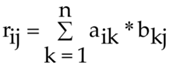Multiply each row by each column then find the sum, and place this sum in the element rij of R

4 of 29

## Matrix multiplication 25 of 29

## Matrix multiplication properties

> if A*B is ok then B*A may not be possible,  A*B ≠ B*A

> Commutative - 2 matricies A, B are said to cummute if and only if A*B = B*A

> Associative - matrix multiplication is associative; A * (B * C) = (A * B) * C = A * B * C

> Distributive - matrix multiplication is distrutive; A * (B + C) = (A * B) + (A * C)

6 of 29

## Matrix Transpose

A transposed is AT

Here the matrix is flipped: rows become columns and columns become rows.

> If R = A then rij = aij.

> If A is size mxn then AT is size nxm

Rules:

Symmetric if  A = AT and skew-symmetric if A =-AT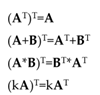7 of 29

## special matricies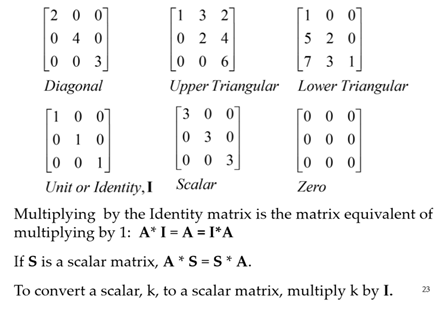8 of 29

## Geometric Transformation

Rotation

New location of object TT = R(Ө) * T where R(Ө)  is the rotation matrix: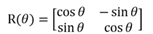Translation

To translate, simply add the value to be translated by to the matrix

Translation and roation

multiply by rotation matrix then add the translation matrix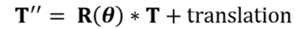9 of 29

## Matrix inverses

Let A be an n x n matrix. If there exists and n x n matrix B such that AB = I = AB we can say A is invertible and call B the matrix inverse of A.

The inverse of matrix A is denoted A-1

A-1 is defined as: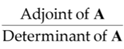A matrix has no inverse if its determinant is 0.

If det(A) ≠ 0, the matrix is invertible – non-singular

If det(A) = 0, then A-1 does not exist - singular

10 of 29

## Matrix determinants, Adjoints, cofactor and minors

The determinant of A is written as det(A) or |A|

Adj(A) is the transpose of the matrix of the cofactors of A.

If Cij is the cofactor of aji then Adj(A) = [Cji] = [Cij]T

Each element Aij of a matrix A has a cofactor Cij = -1(i+j)*Mij, Mij is the determinant of the matrix which is left when row i and column j are removed from A.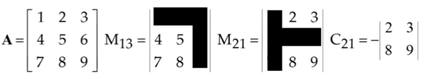11 of 29

## Matrix determinant of 2x2 and 3x3

The determinant of an nxn matrix is the sum of each element in its top row mulitplies by that element's cofactor.

2x2 formula

|A| = (a11 * a22) - (a12 * a21)

3x3 formula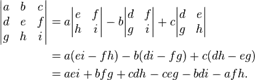12 of 29

## Matrix adjoint 2x2 and 3x3

Adj(A) is the transpose of the matrix of cofactors of A

2x2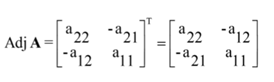3x3

Find the matrix of cofactors and then transpose (swap columns and rows)

13 of 29

## Properties of Determinants

Let A be an n x n matrix and c be scalar. Then,

Det(cA) = cn det(A)

Let A and B be square matricies of the same size. then,

det(AB) = det(A)det(B)

This can be extended to more matricies, provided they are all of the same size:

det(ABCD...) = det(A)det(B)det(C)det(D)....

14 of 29

## Gaussian Elimination and argumented matrix

For a linear equation in matrix form Ax = b, the first step in gaussian elimination is to form the argumented matrix:  Ã = [A|b]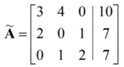There are 3 elementary operations:

• interchange any 2 rows
• multiply (or divide) any row by a non-zero scalar
• Add ( or subtract) a multiple of one row to (or from) another row

When the matrix is in echelon form, gaussian elimination is complete

15 of 29

## Echelon Form

A matrix is in echelon form if:

• Any rows consisting entirely of zeros are the last rows of the matrix
• As you move down the rows of the matrix, the leading entry (the leftmost non-zero entry) moves progressively to the right.
• All entries in a column below a leading entry are zero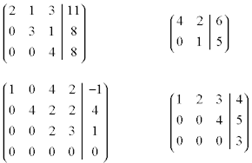16 of 29

## Gauss Jordon method for finding the inverse

First write the argumented matrix in the form [A I]: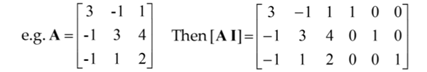Perform elemntary row operations until the matrix is of the form [I B], that is the left half of the matrix is the identity.

Then B, the right half of the matrix will be the inverse of A

A-1 = B

17 of 29

## Cramer's Theorem

An alternative to Gaussian elimination.

Solutions to a linear system A * x = b, where A is an n x n matrix:

x1 = D1/D

x2 = D2/D

xn = Dn/D

where Dk is det|A|, Det <> 0 and Dk is the determinant of the matrix formed by taking A and replacing its kth column with b.

Impracticable in large matricies.

For a 2x2 matrix multiplied by a 2x1 matrix = 2x1 matrix, to find D1 and D2:

Replace the 1st column of A with b and find the determinant. Then replace the 2nd column of with b and find the determinant.

18 of 29

## Number of solutions

No solutions:

2 parallel lines, after gaussian elimination the matrix final line is 0 = a number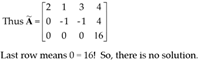Infinite solutions:

2 lines overlap, after gaussian elimination, the matrix final line is 0 = 0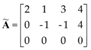19 of 29

## Number of solutions 2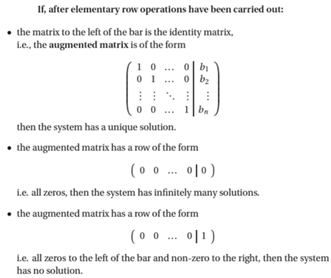20 of 29

## Rank

The rank of a matrix can be used to determine the number of solutions to the equation Ax = b

Definition 1:

> the  number of non zero rows in the argumented matrix when in row echelon form.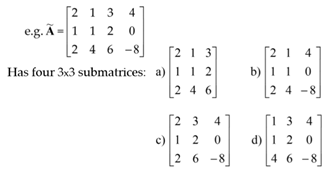Definition 2:

> by determinant, rank of m x n matrix is largest square submatrix whose det ≠ 0. A submatrix of A is a matrix of A minus some rows or columns

.

21 of 29

## Rank and number of solutions

Infinite number of solutions

Det ≠ 0, Rank(Ã) < number of rows

No solutions

Det ≠ 0, Rank(Ã) > Ranks(A)

One solution

Det ≠ 0, Rank(Ã) = Ranks(A)

22 of 29

## Fundamental Theorem of linear systems

If system defined by m-row matrix equation A x = b

The system has solutions only if Rank(A) =Rank(Ã)

• if Rank(A) = m, there is 1 solution
• if Rank(A) < m, there is an infinite number of solutions
23 of 29

## Properties of Rank, homogeneous systems and cramer

Properties of Rank

• The Rank of A is 0 only if A is the zero matrix
• Rank(A) = Rank(AT)
• Elementary row operations don't effect the rank of a matrix.

Homogeneous Systems

• If system defined by Ax = 0 (b=0), system is homogenous.
• A homogenous system always has at least 1 solution, that is a trivial solution, x1 = x2 = ... xn = 0
• A non-trivial solution exists if Rank(A) < m

Cramer's rule

For a homogeneous system:

• If det(A)≠0 the only solution is x = 0 (non-trivial)
• If det(A) = 0, the system has infinite number of non trivial solutions
24 of 29

## Eigenvalues and Eigenvectors

The eigenvalue equation:

Ax = λx

let A be a given n x n matrix. if we can find the non-trivail solution, an n x 1 column vector x and a scalar λ such that Ax = λx then we call λ the eigenvalue and x the associated eignevector.

Here A is nxn matrix, x an nx1 matrix and λ a scalar.

Each (of n) scalar λ satisfying Ax = λx is an eigenvalues of A.

For each λ, an x which satisfies Ax = λx is an eigenvector of A (sometimes labelled Ʌ)

25 of 29

## Eigenvalue and Eigenvector process

To solve for the eigenvalues, λI and the corresponding eigenvectors xi, of an nxn matrix A:

• multiply an nxn identity matrix by the scalar
• subtract the identity matrix multiple from the matrix A
• Find the determinant of the matrix and the difference
• Solve for the values of λ that satisfy the equation det(A-λI)x = 0
• Solve the corresponding vector for each λ
26 of 29

## Eigenvalue and Eigenvector 2x2

1. Find |A - λ*I|, then find the characteristic polynomial and factories to find λ1 and λ2.

2. For each λ (where x is the eigenvector), find the eigenvector, solve (A - λI)x = 0

• for each λi, substitute into A-λI matrix to create new matrix B
• find the argumented matrix of B
• Use Gaussian elimination to get into Echelon form, to get 2 equations.
• Pick 2 values to sibstitute into the equations, e.g. x1 = 1, x1=2....
27 of 29

## Eigenvalue and Eigenvector 3x3

1. find |A-λI| and hence find the characteristic equation

2. solve the characterist equation to find the eigenvalues, this can be one using the integer root theorem:

• look at the constant term and find all the roots. The roots which when substituted into the equation makes LHS = RHS, is a root.
• Hence a factor has been found, divide the equation by this factor.
• Then factorise the last part of the equation to find the next 2 eigenvalues

3. For (A-λI)x=0, find the echelon form matrix of A-λI

4. for the 3 equations find the values for x1, x2 and x3 by giving 1xn a value.

e.g. x1 could = 1

28 of 29

## Complex and Repeated Eigenvalues

The process for finding complex and repeated eigenvalues is the same as finding real eigenvalues.

29 of 29